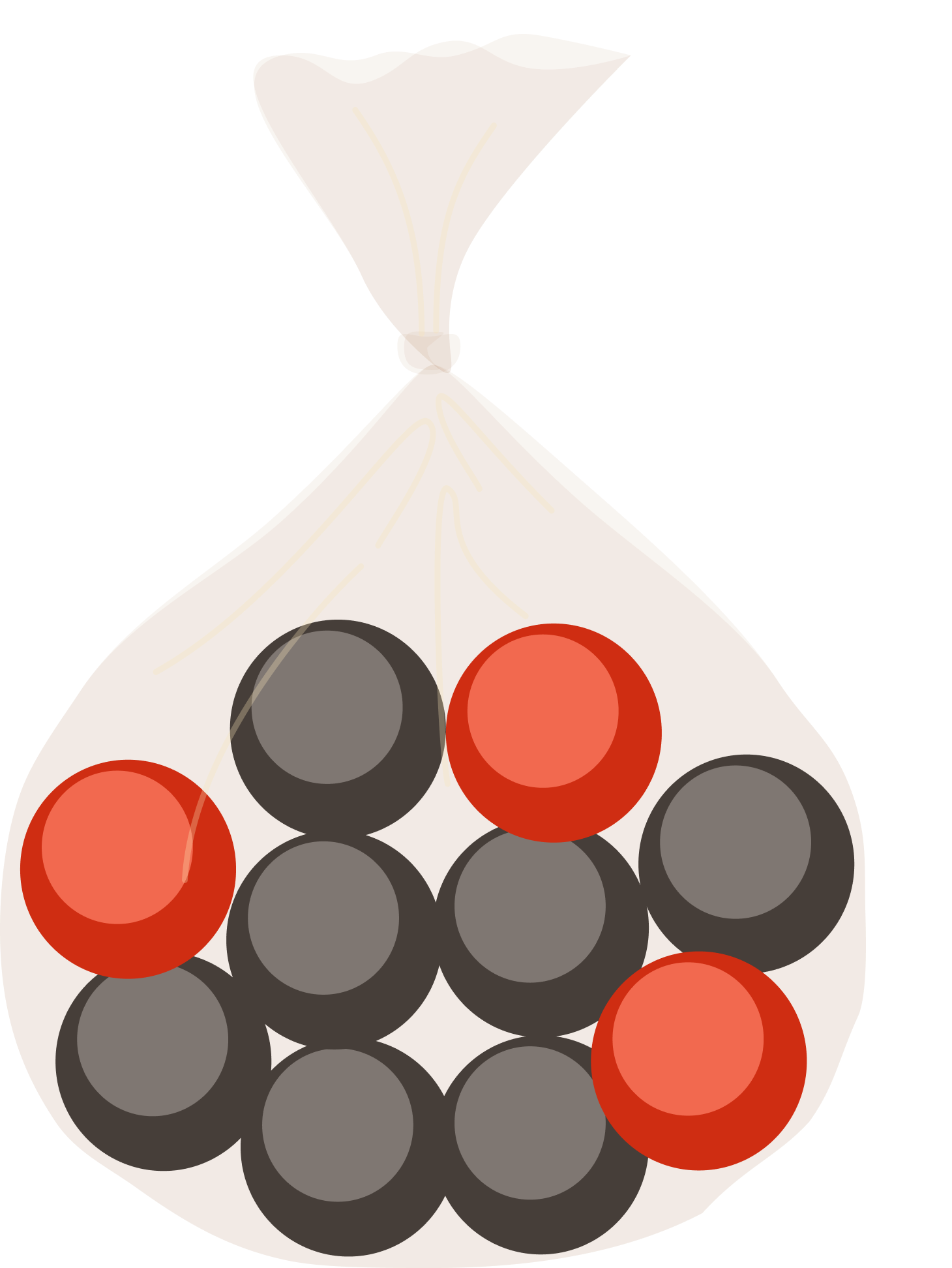# Drawing a Ball!

Probability Level 3A bag contains 3 red and 7 black balls. Two balls are selected at random one-by-one without replacement. If the second selected ball happens to be red, what is the probability that the first ball selected is also red?

The above answer in the form of $\dfrac{a}{b}$, where $a$ and $b$ are co-prime positive integers. Submit your answer as $a + b$

×

Problem Loading...

Note Loading...

Set Loading...